Homework Help Question & Answers

# Part 1- Multiple Choice (30 points) solids and/or hydrogen bonds forces, London dispersion forces, a. Metallic...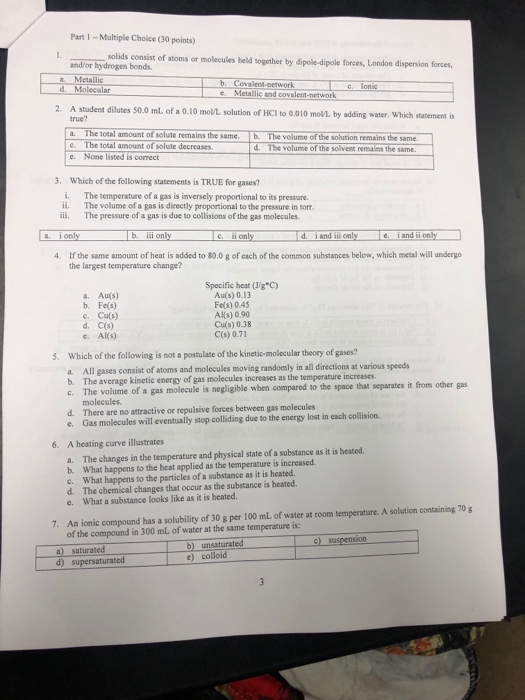Part 1- Multiple Choice (30 points) solids and/or hydrogen bonds forces, London dispersion forces, a. Metallic d. Molecular network c. Metallic and covalent-network 2. A student dilutes 50.0 mL of a 0.10 mon solution of HC? to 0.010 mon by adding water, which statement is true? . The total amount of solute remains the same. b. The volume of the solution remains the same C. The total amount of solute decreases.The volume of the solvent remalins the same d. The volume of the solvent remains the e. None listed is correct 3. Which of the following statements is TRUE for gases? i. The temperature of a gas is inversely proportional to its pressare. ii. The volume of a gas is directly proportional to the pressure in torr iii. The pressure of a gas is due to collisions of the gas molecules a. i only b, ii only d.iand il only i an i only If the same amount of heat is added to 80.0 g of each of the common substances below, which metal will undergo the largest temperature change? 4. Specific heat (Jg°C) Au(s) 0.13 Fe(s) 0.45 AKS) 0.90 Cu(s) 0.38 C(s) 0.71 a. Au(s) b. Fe(s) c. Cu(s) d. C(s) e. Als) Which of the following is not a postulate of the kinetic-molecular theory of gases? 5. All gases consist of atoms and molecules moving randomly in all directions at various speeds b. The average kinetic energy of gas molecules increases as the t emperature increases from other gas The volume of a gas molecule is negligible when compared to the space that separates it molecules. d. There are no attractive or repulsive forces between gas molecules e. Gas molecules will eventually stop colliding due to the energy lost in each collision 6. A heating curve illustrates The changes in the temperature and physical state of a substance as it is heated a. b. What happens to the heat applied as the temperature is increased What happens to the particles of a substance as it is heated. c. d. The chemical changes that occur as the substance is heated. e. What a substance looks like as it is heated An ionic compound has a solubility of 30 g per 100 mL of water at room temperature. A solution containing 70 of the compound in 300 mL of water at the same temperature is: 7. 9) suspension a) saturated d) b) unsaturated e) colloid ated

#### Homework Answers

Answer #1

Solved the first two problems since MCQ type, as per Chegg guidelines ,post one problem per posted question

1) The correct answer is Option d

The molecular bonds will form dipole-dipole interactions, london dispersion and hydrogen bonds

2) The correct answer is Option a

The total amount of solute is irrespective of dilution, since the number of moles of solute will be fixed which is

number of moles of HCl = volume of solution (in L) * molarity = 50/1000 * 0.1 = 0.005 moles

Know the answer?
Your Answer:

#### Post as a guest

Your Name:

What's your source?

#### Earn Coin

Coins can be redeemed for fabulous gifts.

Not the answer you're looking for? Ask your own homework help question. Our experts will answer your question WITHIN MINUTES for Free.
Similar Homework Help Questions
• ### All of the following statements about the kinetic molecular theory of gases are true EXCEPT: A....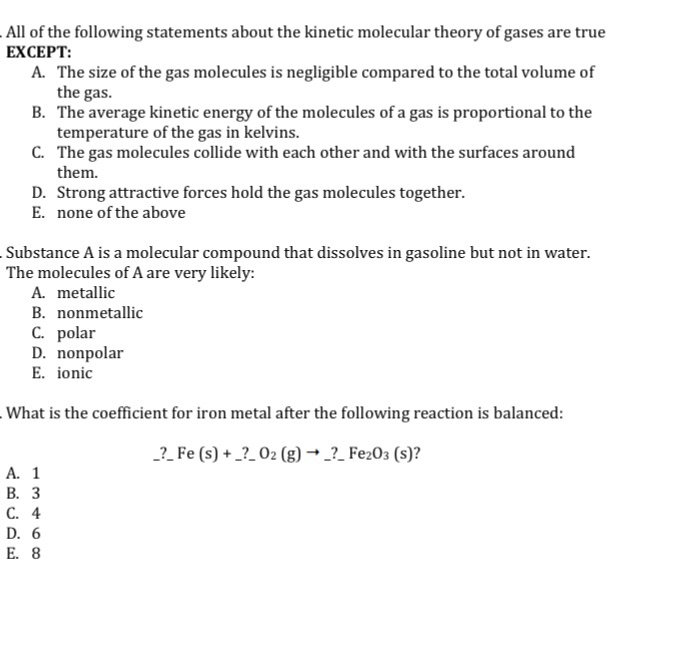All of the following statements about the kinetic molecular theory of gases are true EXCEPT: A. The size of the gas molecules is negligible compared to the total volume of the gas B. The average kinetic energy of the molecules of a gas is proportional to the temperature of the gas in kelvins. C. The gas molecules collide with each other and with the surfaces around them. D. Strong attractive forces hold the gas molecules together. E. none of the...

• ### Problem 4: Read Appendix 2 below (Sec. 1.4.1 of Kasap) and then solve. A metallic back...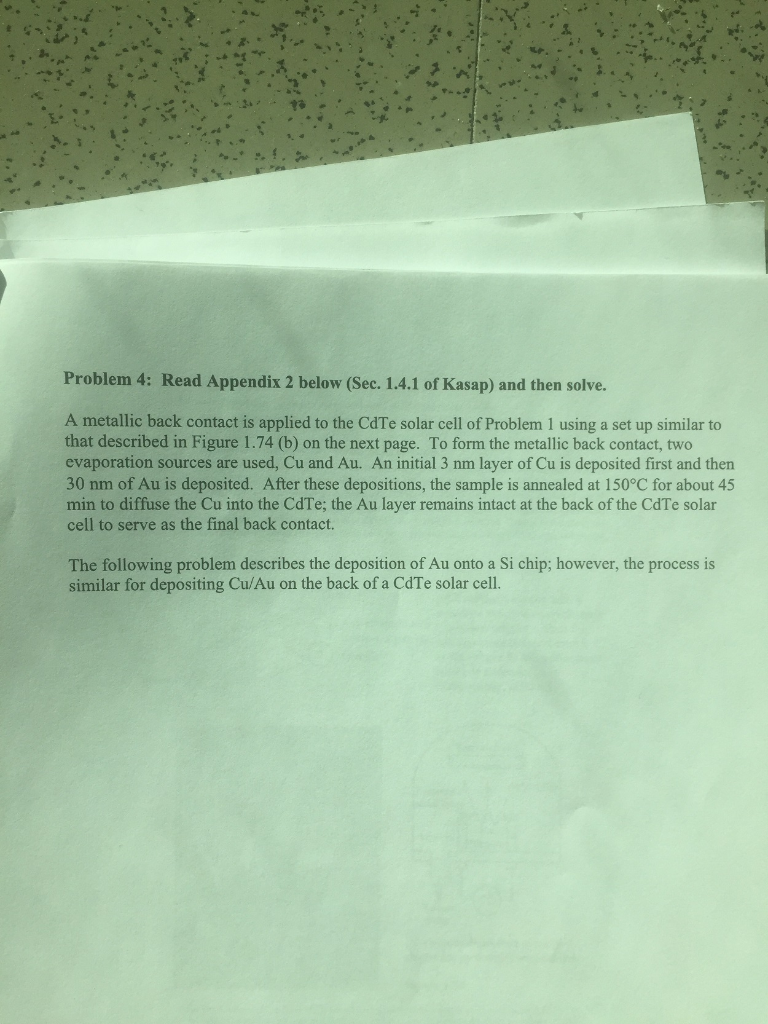Problem 4: Read Appendix 2 below (Sec. 1.4.1 of Kasap) and then solve. A metallic back contact is applied to the CdTe solar cell of Problem 1 using a set up similar to that described in Figure 1.74 (b) on the next page. To form the metallic back contact, two evaporation sources are used, Cu and Au. An initial 3 nm layer of Cu is deposited first and then 30 nm of Au is deposited. After these depositions, the sample...

• ### All of the following statements about the kinetic molecular theory of gases are true EXCEPT: 1....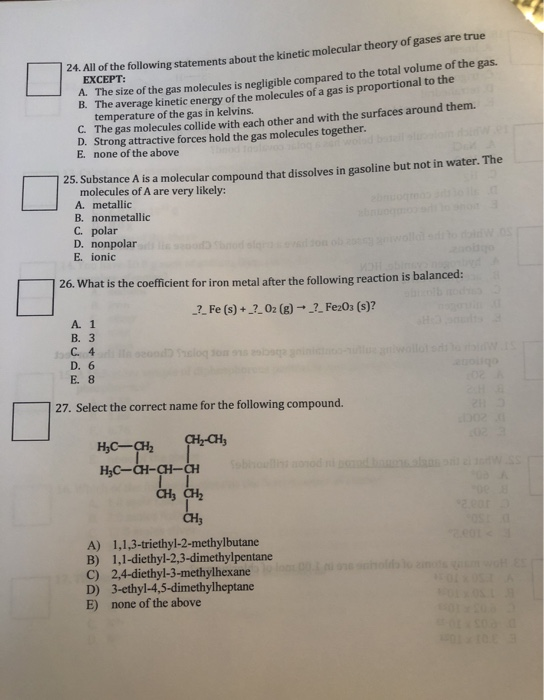All of the following statements about the kinetic molecular theory of gases are true EXCEPT: 1. The size of the gas molecules is negligible compared to the total volume of the gas. B. The average kinetic energy of the molecules of a gas is proportional to the temperature of the gas in kelvins. C. The gas molecules collide with each other and with the surfaces around them. D. Strong attractive forces hold the gas molecules together. E none of the...

• ### Two Part Thermodynamics Multiple Choice Question Q1a. For an ideal gas in an isothermal system the...

Two Part Thermodynamics Multiple Choice Question Q1a. For an ideal gas in an isothermal system the change in .................. is zero A) Heat B) Work C) Entropy D) Internal Energy Q1b. In a piston with insulated external walls there is a movable (insulated)barrier separating the two halves of the piston. The gas in the left chamber is heated. Which of the following is not correct? A) The temperature in the left chamber will increase B) The pressure in both chambers...

• ### 1. Definitions. Fill in the blank with the LETTER of the most appropriate term from the...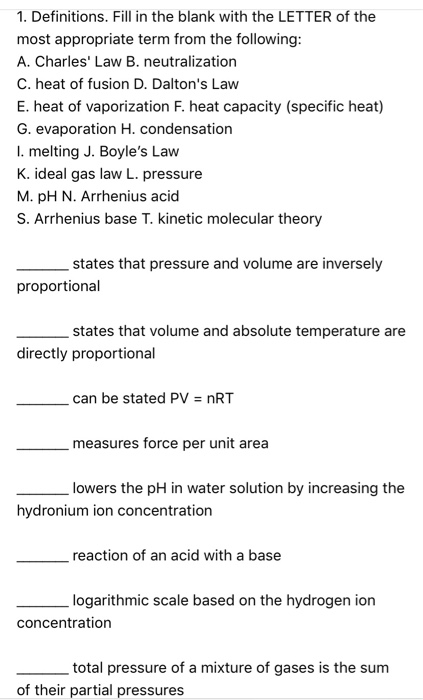1. Definitions. Fill in the blank with the LETTER of the most appropriate term from the following: A. Charles' Law B. neutralization C. heat of fusion D. Dalton's Law E. heat of vaporization F. heat capacity (specific heat) G. evaporation H. condensation I. melting J. Boyle's Law K. ideal gas law L. pressure M.PH N. Arrhenius acid S. Arrhenius base T. kinetic molecular theory states that pressure and volume are inversely proportional states that volume and absolute temperature are directly...

• ### 1,4? Part I: Choose five of these questions. (12 points each) 1. Here is the van...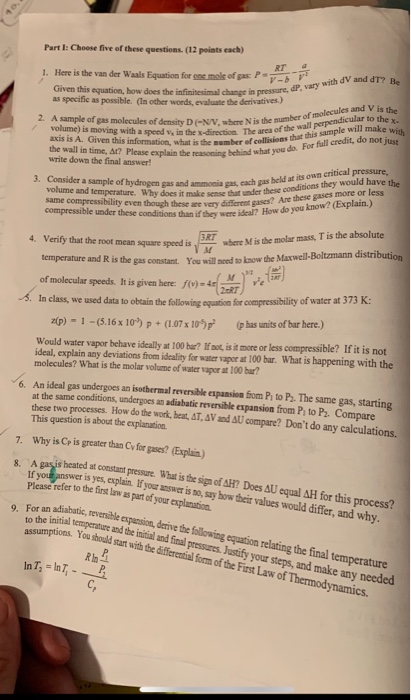1,4? Part I: Choose five of these questions. (12 points each) 1. Here is the van der Waals Equation for one mole of gas P Given this equation, how does the infinitesimal change in pressure, or as specific as possible. (In other words, evaluate the derivatives.) with d V and dT? B e-NV, where is the sity her of molecules and V is the fshe wall perpendicular to the 2. A sample of gas molecules of density N e e...

• ### Which of the following concerning a barometer or manometer is/are true? Pressure is directly proportional to...

Which of the following concerning a barometer or manometer is/are true? Pressure is directly proportional to the height of the liquid used in the device. Pressure is directly proportional to the density of the liquid used in the device. Pressure is independent of the acceleration of gravity. A.1 only B.2 only C.3 only D.1 and 2 E.1, 2, and 3 Which statement is inconsistent with the kinetic theory of an ideal gas? A.Most of the volume occupied by a gas...

• ### one contains helium the other contains the following questions A. The Osygen Balloon B The Helium...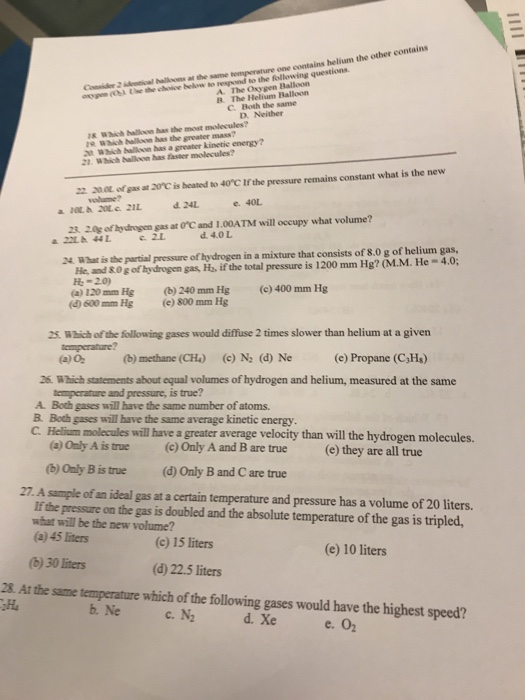one contains helium the other contains the following questions A. The Osygen Balloon B The Helium Balloon C Both the same D. Neither Consider 2 identical halls at the same s Which haloon has the most molecules? 19 Which haillson has the greater mass? t Which balloon has a greater kinetic energy? 2. Wich balon has faster molecules? 22. 20.0L ofgasat arc is heated to 40 C If the pressure remains constant what is the new vlume? e. 40L z3...

• ### What is/are a characteristic/s of an ideal solution? Multiple answers: You can select more than one option...

What is/are a characteristic/s of an ideal solution? Multiple answers: You can select more than one option A) They follow the ideal gas law B) There is no heat exchanged when mixed C) Only solutions with weak intermolecular forces are ideal solutions D) Only solutions with strong intermolecular forces are ideal solutions E) Intermolecular force strengths between solvent and solute particles are similar

• ### For Parts 1 and 2, answer the following multiple choice questions, showing all work to prove...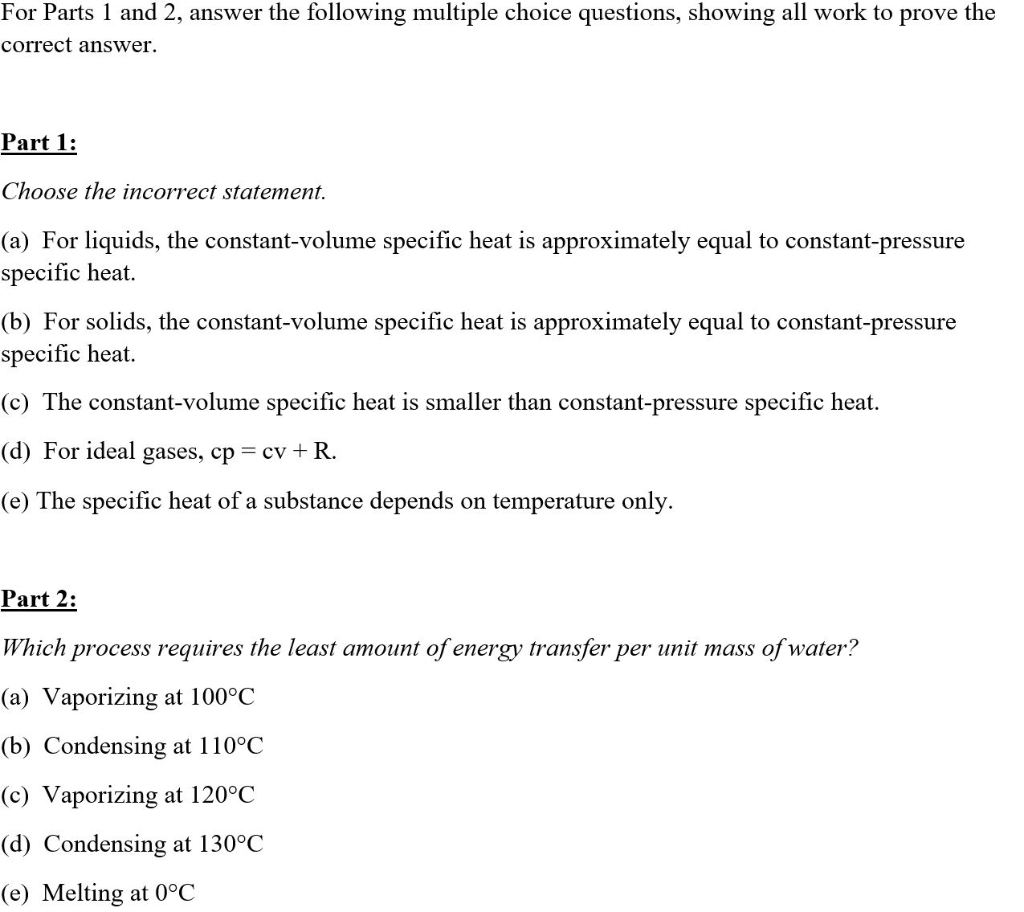For Parts 1 and 2, answer the following multiple choice questions, showing all work to prove the correct answer. Part 1: Choose the incorrect statement. (a) For liquids, the constant-volume specific heat is approximately equal to constant-pressure specific heat. (b) For solids, the constant-volume specific heat is approximately equal to constant-pressure specific heat. (c) The constant-volume specific heat is smaller than constant-pressure specific heat. (d) For ideal gases, cp = cv +R. (e) The specific heat of a substance depends...

Free Homework App

Scan Your Homework
to Get Instant Free Answers
Need Online Homework Help?

Get Answers For Free
Most questions answered within 3 hours.